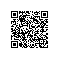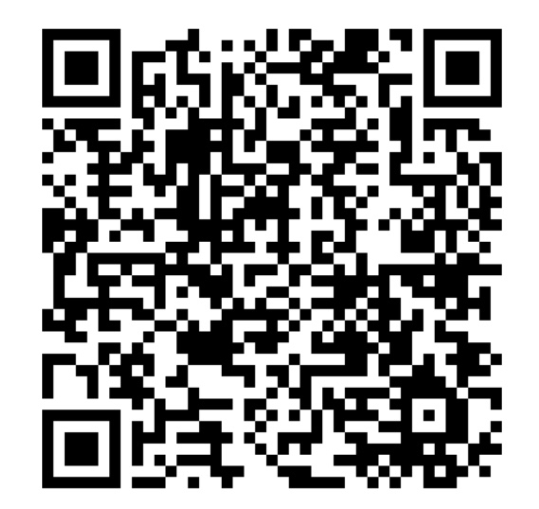# [LeetCode] One Edit Distance 一个编辑距离

Given two strings S and T, determine if they are both one edit distance apart.

1. 两个字符串的长度之差大于1，那么直接返回False

2. 两个字符串的长度之差等于1，那么长的那个字符串去掉一个字符，剩下的应该和短的字符串相同

3. 两个字符串的长度之差等于0，那么两个字符串对应位置的字符只能有一处不同。

class Solution {
public:
bool isOneEditDistance(string s, string t) {
if (s.size() < t.size()) swap(s, t);
int m = s.size(), n = t.size(), diff = m - n;
if (diff >= 2) return false;
else if (diff == 1) {
for (int i = 0; i < n; ++i) {
if (s[i] != t[i]) {
return s.substr(i + 1) == t.substr(i);
}
}
return true;
} else {
int cnt = 0;
for (int i = 0; i < m; ++i) {
if (s[i] != t[i]) ++cnt;
}
return cnt == 1;
}
}
};

class Solution {
public:
bool isOneEditDistance(string s, string t) {
for (int i = 0; i < min(s.size(), t.size()); ++i) {
if (s[i] != t[i]) {
if (s.size() == t.size()) return s.substr(i + 1) == t.substr(i + 1);
else if (s.size() < t.size()) return s.substr(i) == t.substr(i + 1);
else return s.substr(i + 1) == t.substr(i);
}
}
return abs((int)s.size() - (int)t.size()) == 1;
}
};使用钉钉扫一扫加入圈子
+ 订阅